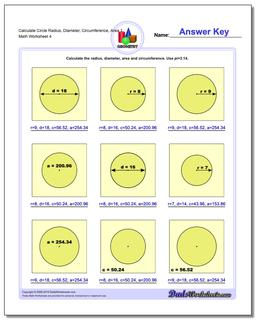# Math Worksheets: Basic Geometry: Basic Geometry: Calculate Circle Radius, Diameter, Circumference, Area 1 (Fourth Worksheet)## Calculate Circle Radius, Diameter, Circumference, Area 1 (Fourth Worksheet)

PropertyValue
DescriptionCalculate Circle Radius, Diameter, Circumference, Area 1: Calculate the radius, diameter, area and circumference given one circle fact. (Fourth Worksheet)
Resource TypeWorksheet